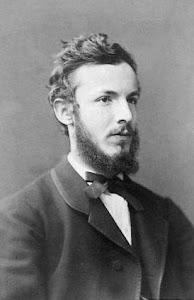### Freedom and truth in mathematics

The very essence of #mathematics is its freedom. (Georg #Cantor)The way we deal with today's numbers in schools is essentially the same manner used by our ancestors Pythagoreans, who saw the numbers as concrete objects, of course, but in a way that prevented them from conceiving the infinity. The only ancient mathematician who approached the infinity was Archimedes, but in the history of mathematics can be considered a fairly unique case of lack of development mainly due to the isolation of the mathematicians at that time and of noticeable difference in quality between the Sicilian and colleagues. In order to return to touch the wall of infinity and use them in a profitable way the Earth had to wait the arrival of Georg Cantor.
The German mathematician actually faced numbers, revolutionizing mathematics, using essentially sets and logic, two tools that enabled him not only to approach, but even manipulate the infinite thanks to the transfinite numbers. Leading his steps was probably the following conviction:
The very essence of mathematics is its freedom.
According to Daniel Bonevac, this veritable mantra, written in 1883, is emblematic of the Cantor's libertarian approach to mathematics. With this milestone, Bonevac try to write a theory of mathematical truth, in order to explain some facts more or less established:
1) that the mathematical statements are either necessarily true or necessarily false;
2) that mathematical truth is derived primarily from logical truth;
3) that the existence in mathematics involves a kind of modality, which requires only the consistency or the constructability.
The construction of the theory builds on the mathematical language $L$ of Zermelo-Fraenkel set theory, which is appropriately extended in a new language, said $L'$, containing a set of constants metalinguistic statements (but not necessarily finite). Given this extension, then any predicate in $L$ will be a predicate in $L'$ not with constants.
The last step is the definition of a parametric model $M$, defined as a pair of applications: the first associates to each atomic predicate of $L$ a truth value; the second is in turn a set of applications that can be called rules of the game, showing explicitly, if it were needed, that the modern game theory is simply a some kind of logical application.
The idea of ​​the approach is quite simple. Take first the phrase "There is a pebble in my shoe". Its degree of truth depends by the inclusion of the word pebble in our vocabulary, and so you need to extend the language to include the word, or in other words it is possible, under the circumstances, the restriction in situations in which it is true that "There is a pebble in my shoe".
On the other hand "Some flying horse is named Pegasus" is true in the case of a mythological game, false in the case of a biological game.
In particular the latter situation suggests Bonevac to adopt an approach that is as inclusive as possible, or that it does not exclude any of the possible games: in this way you get what Bonevac calls the semantics of open models.
The mathematics that emerges from this semantic discussion contains within it the Godel's incompleteness theorems, showing that they are inseparable from mathematics itself, but also show themselves to be more natural than amazing. Bonevac shows also how the concept of truth is necessary to mathematics, which becomes explicitly dependent from logic. On the other hand within this semantic not only the continuum hypothesis of Cantor is unprovable, but, together with the axiom of choice, it is false.
We can only assume that, as with many cantorian logicians, the initial goal of Bonevac was to build an axiomatic system in which the continuum hypothesis were true, but even if this goal was not achieved, in my view he has instead proofed something that we usually take to established (and the denial of which sometimes becomes a way to challenge our stakeholders mathematicians), or that the math does not lie.
Bonevac D. (1983). Freedom and truth in mathematics, Erkenntnis, 20 (1) 93-102. DOI: http://dx.doi.org/10.1007/bf00166496 (pdf)